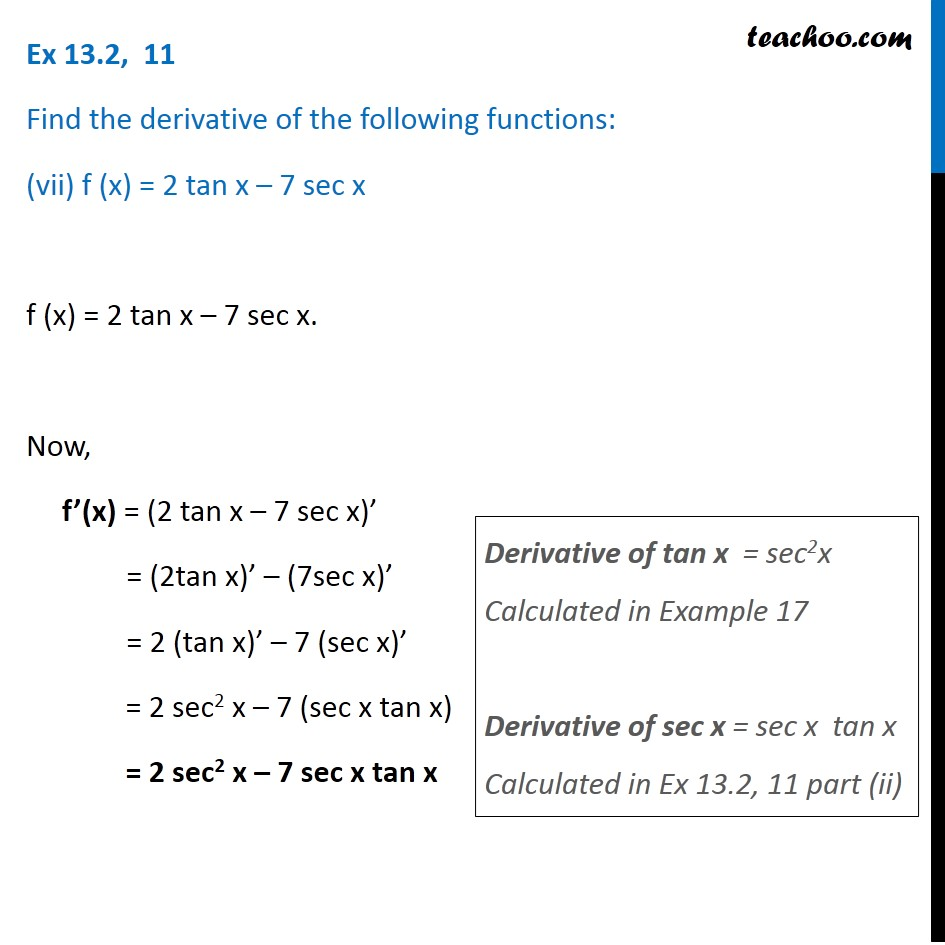Ex 13.2

Chapter 13 Class 11 Limits and Derivatives
Serial order wiseIntroducing your new favourite teacher - Teachoo Black, at only ₹83 per month

### Transcript

Ex 13.2, 11 Find the derivative of the following functions: (vii) f (x) = 2 tan x – 7 sec x f (x) = 2 tan x – 7 sec x. Now, f’(x) = (2 tan x – 7 sec x)’ = (2tan x)’ – (7sec x)’ = 2 (tan x)’ – 7 (sec x)’ = 2 sec2 x – 7 (sec x tan x) = 2 sec2 x – 7 sec x tan x Derivative of tan x = sec2x Calculated in Example 17 Derivative of sec x = sec x tan x Calculated in Ex 13.2, 11 part (ii)